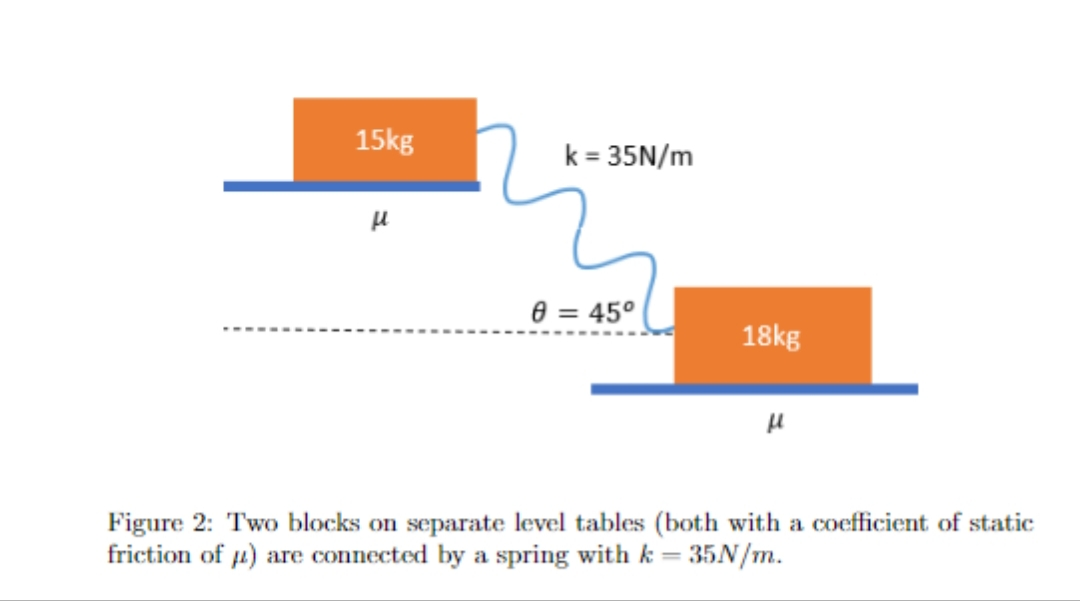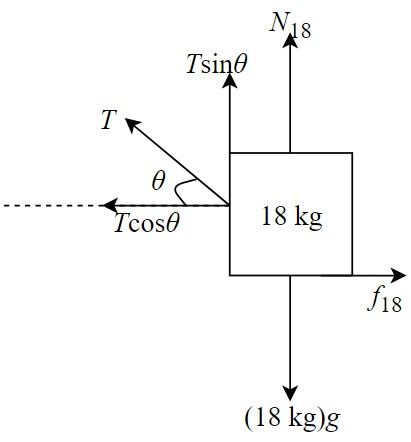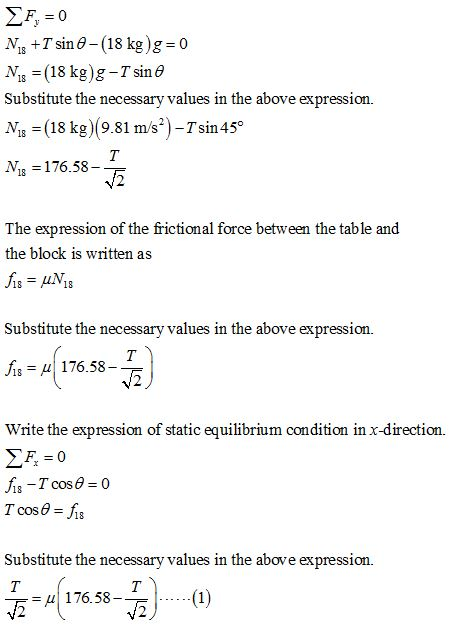# 15kgk = 35N/m8 = 45°18kgFigure 2: Two blocks on separate level tables (both with a coefficient of staticfriction of 4) are connected by a spring with k = 35N/m.

Question
47 views

2. Two blocks on separate level tables are connected by a spring as shown, How much is the spring stretched from its natural length if the system is at rest?help_outlineImage Transcriptionclose15kg k = 35N/m 8 = 45° 18kg Figure 2: Two blocks on separate level tables (both with a coefficient of static friction of 4) are connected by a spring with k = 35N/m. fullscreen
check_circle

Step 1

Consider the tension in the spring to be T, N18 be the reaction of the block with 18 kg mass and f18 be the frictional force between the block and the table. Draw the free-body diagram of the block with the mass of 18 kg.Step 2

Write the expression of static equilibrium condition in the y-direction.Step 3

Understand that the blocks are lying at parallel surfaces to each other thus the spring also makes an angle of 45° with the horizontal.

Consider N15 to be the reaction of the block with 15 kg mass and f15 be the frictional force between the block and the table. Draw the free-body diagram of the block...

### Want to see the full answer?

See Solution

#### Want to see this answer and more?

Solutions are written by subject experts who are available 24/7. Questions are typically answered within 1 hour.*

See Solution
*Response times may vary by subject and question.
Tagged in